Function Repository Resource:

# ToWellKnownText

Convert graphics primitives to well-known text (WKT)

Contributed by: Anton Antonov
 ResourceFunction["ToWellKnownText"][arg] Converts graphics primitives in arg into Well-known text strings.

## Details

ResourceFunction["ToWellKnownText"] converts graphics primities Point, Line, Polygon into Well-Known Text (WKT) strings.
Point[{x_,y_, ___}] is converted into a string that starts with "POINT".
Point[{p1,p2,p3,..}] is converted into a string that starts with "MULTIPOINT".
Line[{p1, p2, …}] is converted to a string that starts with "LINE".
Line[{{p11, p12, …}, {p21, p22, …}, …}] is converted to a string that starts with "MULTILINESTRING".
Polygon[{p1,p2,}] is converted to a string that starts with "POLYGON".
Polygon[{{p11, p12, …}, {p21, p22, …}, …}] is converted to a string that starts with "MULTIPOLYGON".
Lists of graphics primitives are converted to WKT strings that start with "GEOMETRYCOLLECTION".

## Examples

### Basic Examples (2)

Here a graphics specification of three points is converted into a WKT string:

 In:=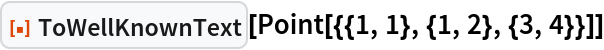Out=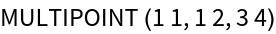Here a multi-element graphics specification is converted into a WKT string:

 In:=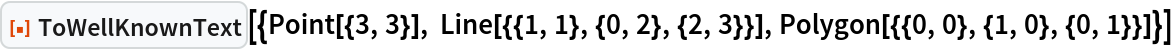Out=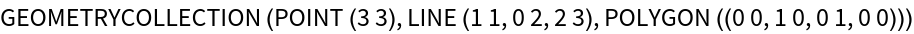### Scope (7)

Over a single point:

 In:=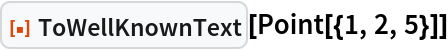Out=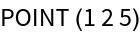Over multiple points:

 In:=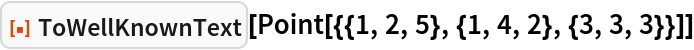Out=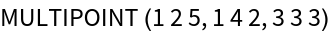Over a line:

 In:=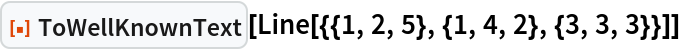Out=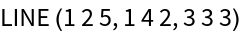Over multiple lines:

 In:=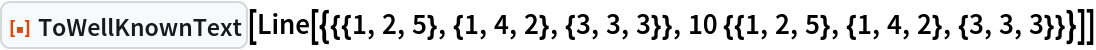Out=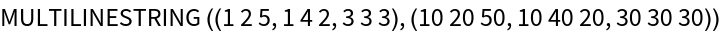Over a polygon:

 In:=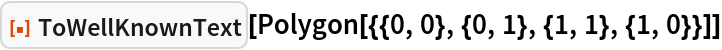Out=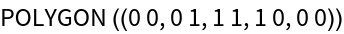Over multiple polygons:

 In:=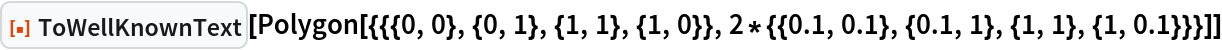Out=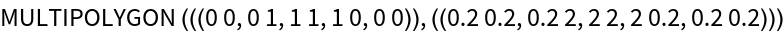Over a collection of polygons and lines:

 In:=Out=### Properties and Relations (2)

Here is multi-element graphics:

 In:=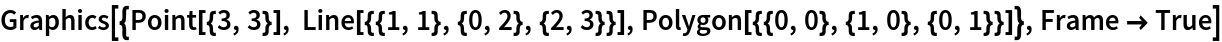Out=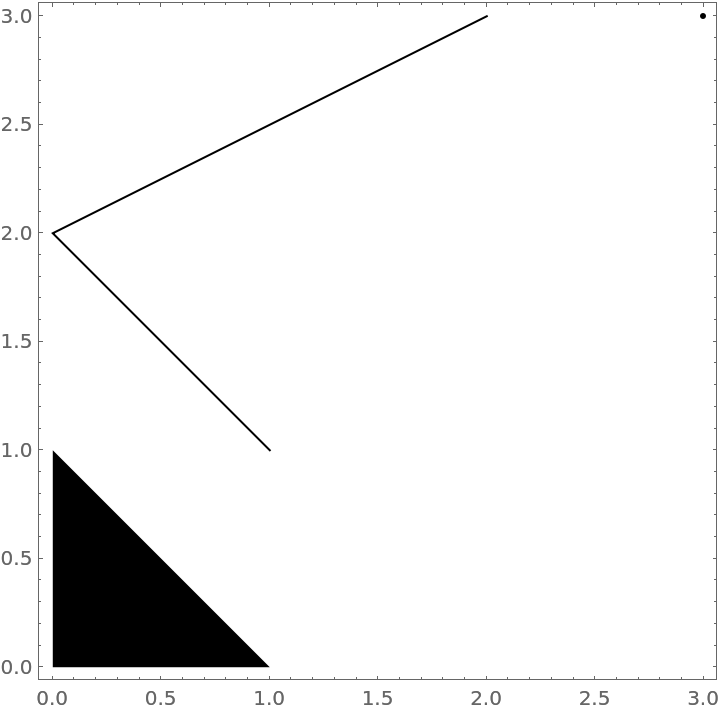Here is the corresponding WKT string:

 In:=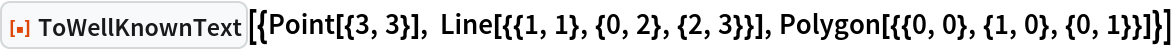Out=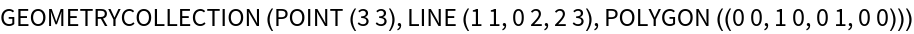Multi-polygon specification:

 In:=Out=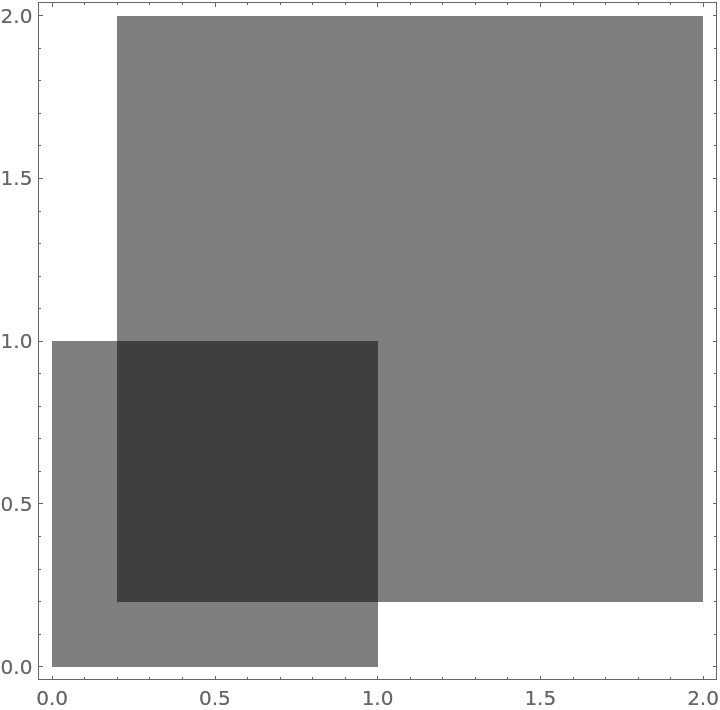Here is the corresponding WKT string:

 In:=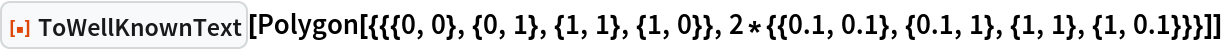Out=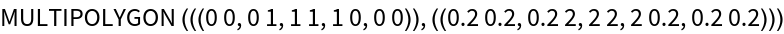### Possible Issues (2)

ToWellKnownText does not check the dimensions of the given graphics specs.

Well-known Text (WKT) specifications allow for representation of polygons with holes. For example:

POLYGON ((35 10, 45 45, 15 40, 10 20, 35 10), (20 30, 35 35, 30 20, 20 30))

The function ToWellKnownText does not make WKT strings for polygons-with-holes.

Anton Antonov

## Version History

• 1.0.0 – 12 September 2022

## Author Notes

Well-known Text (WKT) is used a lot in Geo-computations. A closely related format is Well-known Binary (WKB). WKT and WKB are often converted to GeoJSON.